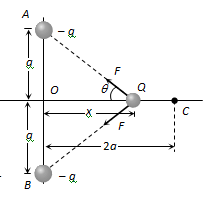# Two equal negative charge – q are fixed at the fixed points (0,a) and (0.-a) on the Y-axis. A positive charge Q is released from rest at the point (2a, 0) on the X-axis. The charge Q willOption 1) Execute simple harmonic motion about the origin Option 2) Move to the origin and remain at rest  Option 3) Move to infinity Option 4) Execute oscillatory but not simple harmonic motion

P Plabita

As we learnt,

Charged Circular ring -

- wherein

By symmetry of problem the components of force on Q due to charges at A and B along y-axis will cancel each other while along x-axis will add up and will be along CO. Under the action of this force charge Q will move towards O. If at any time charge Q is at a distance x from O. Net force on charge Q

As the restoring force Fnet is not linear, motion will be oscillatory (with amplitude 2a) but not simple harmonic.

Option 1)

Execute simple harmonic motion about the origin

Option 2)

Move to the origin and remain at rest

Option 3)

Move to infinity

Option 4)

Execute oscillatory but not simple harmonic motion

Exams
Articles
Questions